# A.y=flexside is revealed and the resort=0x** Mand the volume B Find the surface area ify =...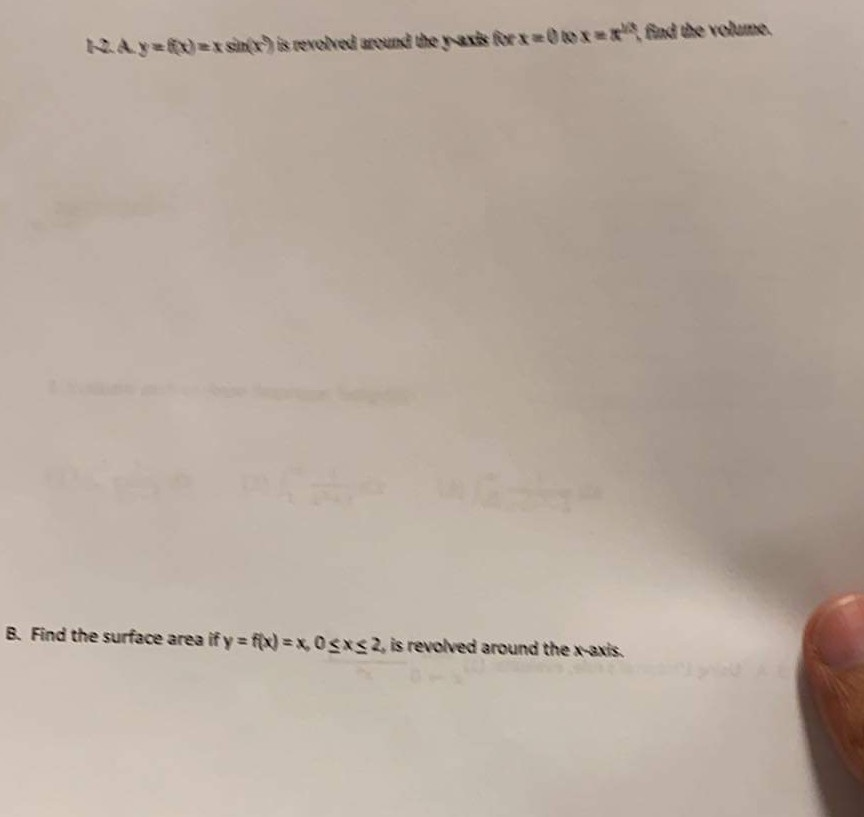A.y=flexside is revealed and the resort=0x** Mand the volume B Find the surface area ify = fx)=x OSXS 2. is revolved around the exis

3) We have given y=f(x)=xsin(x^3) and x=0 to (pi)1/3

by using shell method

we know that the volume of the solid around y-axis is integration of (x=a to b)((2pi)*r*h)dx

we have radius r=x and height h=xsin(x^3) and thickness dx

So Volume V= integration of (x=0 to (pi)1/3)((2pi)*x*xsin(x^3) )dx

=integration of (x=0 to (pi)1/3)((2pi)x2sin(x^3) )dx

substitute u=x^3,du=3x^2dx implies x^2dx=du/3

=(2pi)* integration of (x=0 to (pi)1/3)(sin(u))(du/3)

=(2pi)/3 *integration of (x=0 to (pi)1/3)(sin(u))du

=(2pi)/3*[-cos(u)] from x=0 to (pi)1/3

resubstitute u=x^3

=(2pi)/3*[-cos(x^3)] from x=0 to (pi)1/3

=(2pi)/3*[-cos(((pi)1/3)^3)-(-cos(0))]

=(2pi)/3*[-cos(pi)+1]

=(2pi)/3*[1+1]

=(4*pi)/3

Volume V=(4*pi)/3

#### Earn Coin

Coins can be redeemed for fabulous gifts.

Similar Homework Help Questions
• ### 1. The unbounded plan ar region bet ween the z-axis and the curve yis revolved about the r2 1 T-axis (a) Find the volume of the resulting solid of revolution b) Does the solid have faite surface area...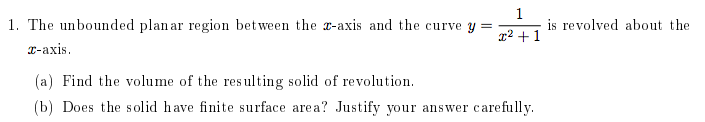1. The unbounded plan ar region bet ween the z-axis and the curve yis revolved about the r2 1 T-axis (a) Find the volume of the resulting solid of revolution b) Does the solid have faite surface area? Justify sour answer carefilly 1. The unbounded plan ar region bet ween the z-axis and the curve yis revolved about the r2 1 T-axis (a) Find the volume of the resulting solid of revolution b) Does the solid have faite surface area?...

• ### Find the area of the surface generated when the given curve is revolved about the given...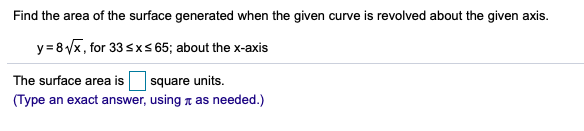Find the area of the surface generated when the given curve is revolved about the given axis. y 8x, for 33sxs 65; about the x-axis The surface area is square units. (Type an exact answer, using a as needed.) Find the area of the surface generated when the given curve is revolved about the given axis. y 8x, for 33sxs 65; about the x-axis The surface area is square units. (Type an exact answer, using a as needed.)

• ### 1) 2) Find the surface area of the volume generated when the curve y = Vx...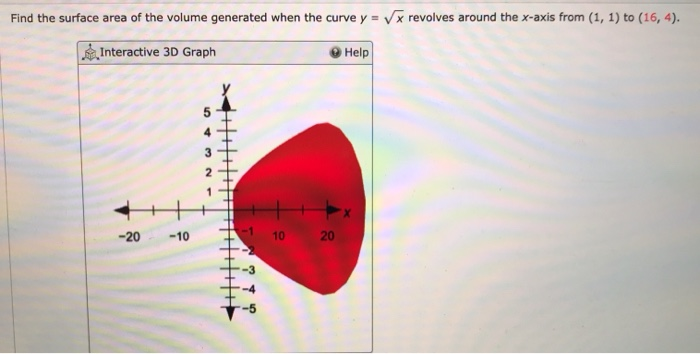1) 2) Find the surface area of the volume generated when the curve y = Vx revolves around the x-axis from (1, 1) to (16, 4). Interactive 3D Graph Help -20 -10 10 20 2-3 Calculate the arc length over the given interval. y : **?- in(x), (1,7) x

• ### 1. The area between the part of the curve-6x 8 above the x-axis and the x-axis itself is 2. The a...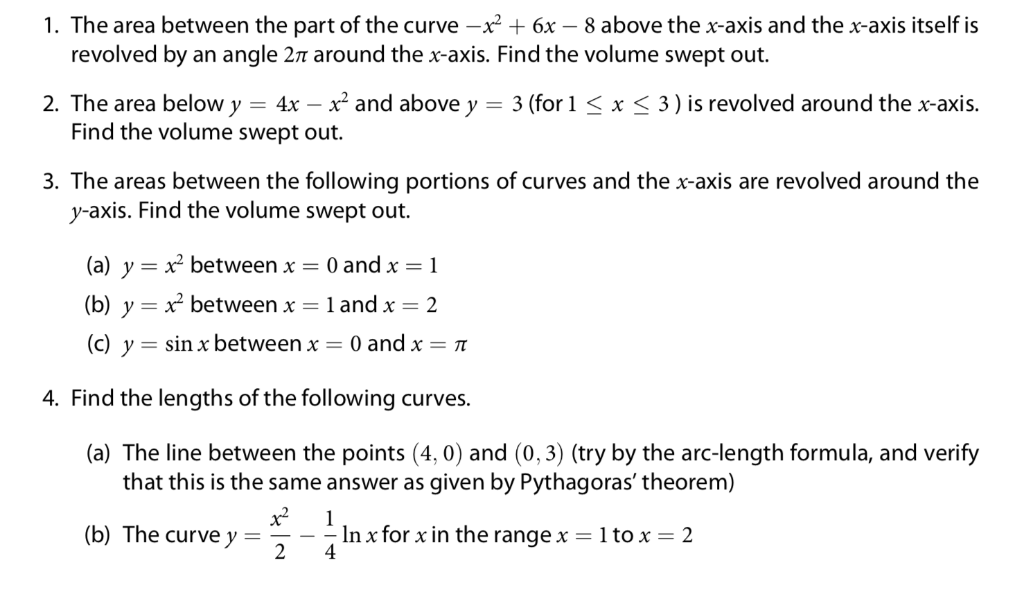1. The area between the part of the curve-6x 8 above the x-axis and the x-axis itself is 2. The area below y 4x -x and above y 3 (for1 xS 3) is revolved around the x-axis. 3. The areas between the following portions of curves and the x-axis are revolved around the revolved by an angle 2π around the x-axis. Find the volume swept out. Find the volume swept out. y-axis. Find the volume swept out. (a) y- betweenx...

• ### Find the area of the surface obtained by revolving the curre ya 83 osxs 2, about...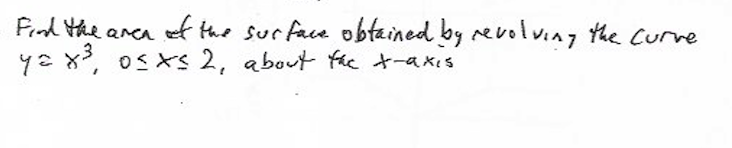Find the area of the surface obtained by revolving the curre ya 83 osxs 2, about the t-axis

• ### 3. Graph the region bounded by the parametric curve x cost and y = et where 0 t Find the length of the curve. b. Fi...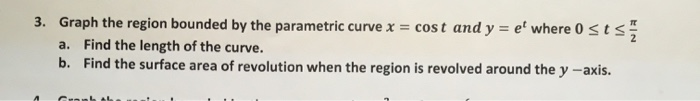3. Graph the region bounded by the parametric curve x cost and y = et where 0 t Find the length of the curve. b. Find the surface area of revolution when the region is revolved around the y -axis. a. 3. Graph the region bounded by the parametric curve x cost and y = et where 0 t Find the length of the curve. b. Find the surface area of revolution when the region is revolved around the y...

• ### Find the area of the surface generated when the given curve is revolved about the x-axis....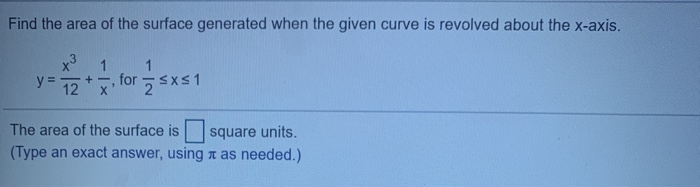Find the area of the surface generated when the given curve is revolved about the x-axis. x3 1 1 y = + 12 for 2 sxs1 х The area of the surface is square units. (Type an exact answer, using it as needed.)

• ### Find the area of the surface generated when the given curve is revolved about the x-axis....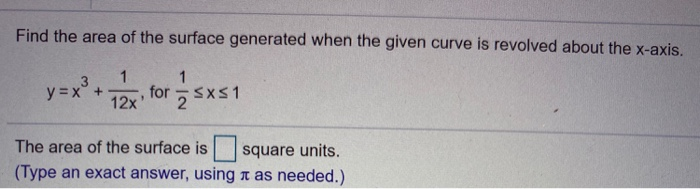Find the area of the surface generated when the given curve is revolved about the x-axis. 1 y = x² + for SXS1 9 12x The area of the surface is square units. (Type an exact answer, using it as needed.)

• ### Find the area of the surface generated when the given curve is revolved about the given...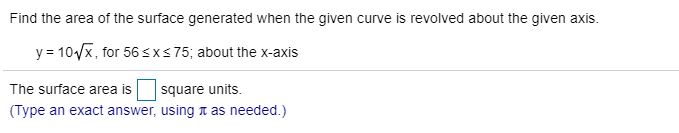Find the area of the surface generated when the given curve is revolved about the given axis. y = 107x, for 565x375; about the x-axis The surface area is square units. (Type an exact answer, using a as needed.)

• ### Find the area of the region enclosed by the curves: x = -sec^2 y, x = sec^2 y, y = 0, y = pi/4 Using the method of cylindrical shells to find the volume of the solid that results when the region enclo...

Find the area of the region enclosed by the curves: x = -sec^2 y, x = sec^2 y, y = 0, y = pi/4 Using the method of cylindrical shells to find the volume of the solid that results when the region enclosed by the curves is revolved around the y axis. y = sqrt (x+1), y = 1, x = 1 y = 3 sqrt x, y =0, x =1 Find the volume of the solid that results when...